# What is graph vulnerability and robustness?

First mentioned as early as the 1970’s, network robustness has a rich and diverse history spanning numerous fields of engineering and science. While the fields of study are diverse, they are linked by a common definition, defined as a

measure of a network’s ability to continue functioning when part of the network is naturally damaged or targeted for attack.

The study of network robustness is a critical tool in the characterization and understanding of complex interconnected systems such as transportation, infrastructure, communication, and computer networks. Through analyzing and understanding the robustness of these networks we can: (1) quantify network vulnerability and robustness, (2) augment a network’s structure to resist attacks and recover from failure, and (3) control the dissemination of entities on the network (e.g., viruses, propaganda).

For example, to minimize the ability of viruses to spread we can monitor (remove) nodes in the graph to reduce graph connectivity. On the other hand, if want to maximize network diffusion e.g., marketing campaign, we can use defense techniques like edge rewiring or addition to increase graph connectivity. In Figure 3 below, we highlight the SIS infectious disease model and how TIGER’s defense techniques can help contain a simulated outbreak.

Here we focus on dissemination of network entities, which attempts to capture the underlying mechanism enabling network propagation. In order to augment the diffusion process, TIGER leverages multiple defense techniques for use with two prominent diffusion models: the flu-like susceptible-infected-susceptible (SIS) model, and the vaccinated-like susceptible-infected-recovered (SIR) model.

To help users visualize the dissemination process, we enable them to create visuals like Figure 1, where we run an SIS computer virus simulation on the Oregon-1 Autonomous System network. The top of Figure 1 shows the virus progression when defending 5 nodes selected by Netshield. By time step 1000, the virus has nearly died out. The bottom of Figure 3 shows that the virus remains endemic without defense.

# What tools are available to study network robustness?

While significant research has been devoted to studying network vulnerability and robustness, no comprehensive open-source toolbox currently exists to assist researchers and practitioners in this important topic. This lack of available tools hinders reproducibility and examination of existing work, development of new research, and dissemination of new ideas.

We believe a unified and easy-to-use software framework is key to standardizing the study of network robustness, helping accelerate reproducible research and dissemination of ideas. As such, we developed TIGER, the first open-sourced Python toolbox for evaluating network vulnerability and robustness of graphs. TIGER contains 22 graph robustness measures with both original and fast approximate versions when possible; 17 failure and attack mechanisms; 15 heuristic and optimization based defense techniques; and 4 simulation tools. To the best of our knowledge, this makes TIGER the most comprehensive open-source framework for network robustness analysis to date.

# Robustness Measures

TIGER contains 22 robustness measures, grouped into one of three categories depending on whether the measure utilizes the graph, adjacency, or Laplacian matrix. In Table 1, we highlight each implemented measure, the category it belongs to (graph, adjacency, Laplacian), and its application to network robustness.

For detailed description and discussion of all 22 measures, we refer the reader to the arXiv paper and Github page.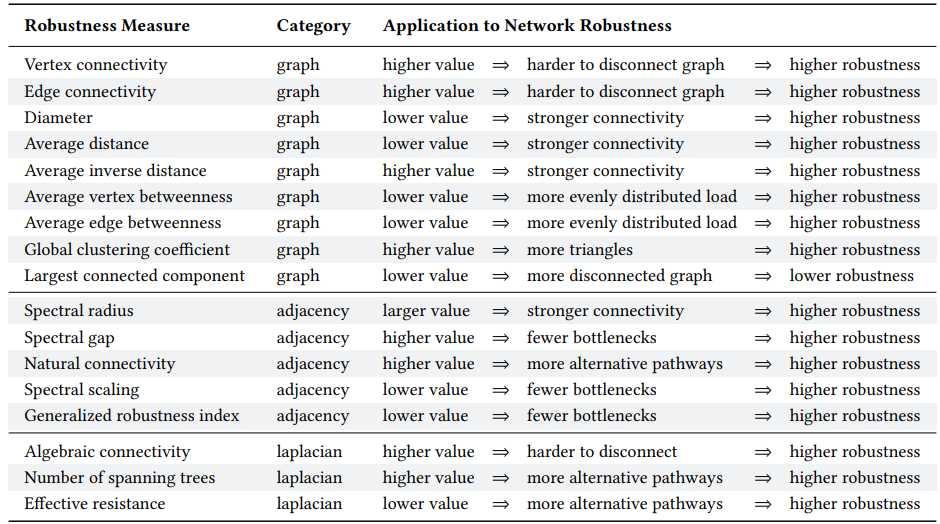Table 1. Comparison of TIGER robustness measures. Measures are grouped based on whether they use the graph, adjacency or Laplacian matrix. For each measure, we describe it’s application to measuring robustness.

# Example Robustness Measures

We select 3 robustness measures, one from each of the three categories, to extensively discuss.

1. Average vertex betweenness (Equation 1) of a graph G=(V, E),where V is the set of vertices and E is the set of graph edges, is the summation of vertex betweenness bᵥ for every node u∈ V, where vertex betweenness for node u is defined as the number of shortest paths that pass through u out of the total possible shortest paths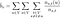Equation 1. Average vertex betweenness measures the average load on vertices in the network. The smaller the average the more robust the network, since load is more evenly distributed across nodes.

where nₛₜ(u) is the number of shortest paths between s and t that pass through u and nₛₜ is the total number of shortest paths between s and t. Average vertex betweenness has a natural connection to graph robustness since it measures the average load on vertices in the network. The smaller the average the more robust the network, since load is more evenly distributed across nodes.

2. Spectral scaling (Equation 2) indicates if a network is simultaneously sparse and highly connected, known as “good expansion” (GE). Intuitively, we can think of a network with GE as a network lacking bridges or bottlenecks. In order to determine if a network has GE, you can combine the spectral gap measure with odd subgraph centrality SC(odd), which measures the number of odd length closed walks a node participates in. Formally, spectral scaling is defined as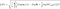Equation 2. Spectral scaling measures the number of bridges and bottlenecks in a graph, the higher the value, the more easily separated the graph (low robustness).

The closer ξ is to zero, the better the expansion properties and the more robust the network. Typically, a network is considered to have GE if ξ< 0.01.

3. Effective resistance (Equation 3) views a graph as an electrical circuit where an edge (i, j) corresponds to a resister of r(i, j) = 1 Ohm and a node i corresponds to a junction. As such, the effective resistance between two vertices i and j, denoted R(i, j), is the electrical resistance measured across i and j when calculated using Kirchoff’s circuit laws. Extending this to the whole graph, we say the effective graph resistance R is the sum of resistances for all distinct pairs of vertices. Klein and Randic proved this can be calculated based on the sum of the inverse non-zero Laplacian eigenvalues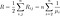Equation 3. Effective resistance measure how well connected a network is, where a smaller value indicates a more robust network.

As a robustness measure, effective resistance measures how well connected a network is, where a smaller value indicates a more robust network. In addition, the effective resistance has many desirable properties, including the fact that it strictly decreases when adding edges, and takes into account both the number of paths between node pairs and their length.

# Network Attacks

There are two primary ways a network can become damaged — (1) natural failure and (2) targeted attack. Natural failures typically occur when a piece of equipment breaks down from natural causes. In the study of graphs, this would correspond to the removal of a node or edge in the graph. While random network failures regularly occur, they are typically less severe than targeted attacks. In contrast, targeted attacks carefully select nodes and edges in the network for removal in order to maximally disrupt network functionality. As such, we focus the majority of our attention to targeted attacks.

We cover one type of attack below, for a detailed description and discussion of all 10 attacks, we refer the reader to the arXiv paper and Github page.

In Figure 2 we simulate an attack on the Kentucky KY-2 water distribution network. The network starts under normal conditions (far left), and at each step an additional node is removed by the attacker (red nodes).
After removing only 13 of the 814 nodes, the network is split into two separate regions. By step 27, the network splits into four disconnected regions. In this simulation, and in general, attack strategies rely on node and edge centrality measures to identify candidates.

# Network Defenses

The same network centrality measures that are effective in attacking a network are important to network defense (e.g., degree, betweenness, PageRank, eigenvector, etc.). In fact, if an attack strategy is known a priori, node monitoring can largely prevent an attack altogether.

We categorize defense techniques based on whether they operate heuristically, modifying graph structure independent of a robustness measure, or by optimizing for a particular robustness measure. Within each category, a network can be defended i.e., improve its robustness by — (1) edge rewiring, (2) edge addition, or (iii) node monitoring.

Edge rewiring is considered a low cost, less effective version of edge addition. On the other hand, edge addition almost always provides stronger defense. Node monitoring provides an orthogonal mechanism to increase network robustness by monitoring (or removing) nodes in the graph. This has an array of applications, including: (i) preventing targeted attacks, (ii) mitigating cascading failures, and (iii) reducing the spread of network entities.

We evaluate the effectiveness of 5 edge defenses on the Kentucky KY-2 water distribution network (see Figure 2), averaged over 10 runs. The network is attacked by removing 30 of the most “important” nodes using a degree centrality attack strategy , and the success of each defense is measured based on how it can reconnect the network by adding or rewiring edges in the network (higher is better). Based on Figure 2, we identify three key observations — (i) preferential edge addition performs the best; (ii) edge addition, in general, outperforms rewiring strategies; and (iii) random neighbor rewiring typically performs better than the other rewiring strategies.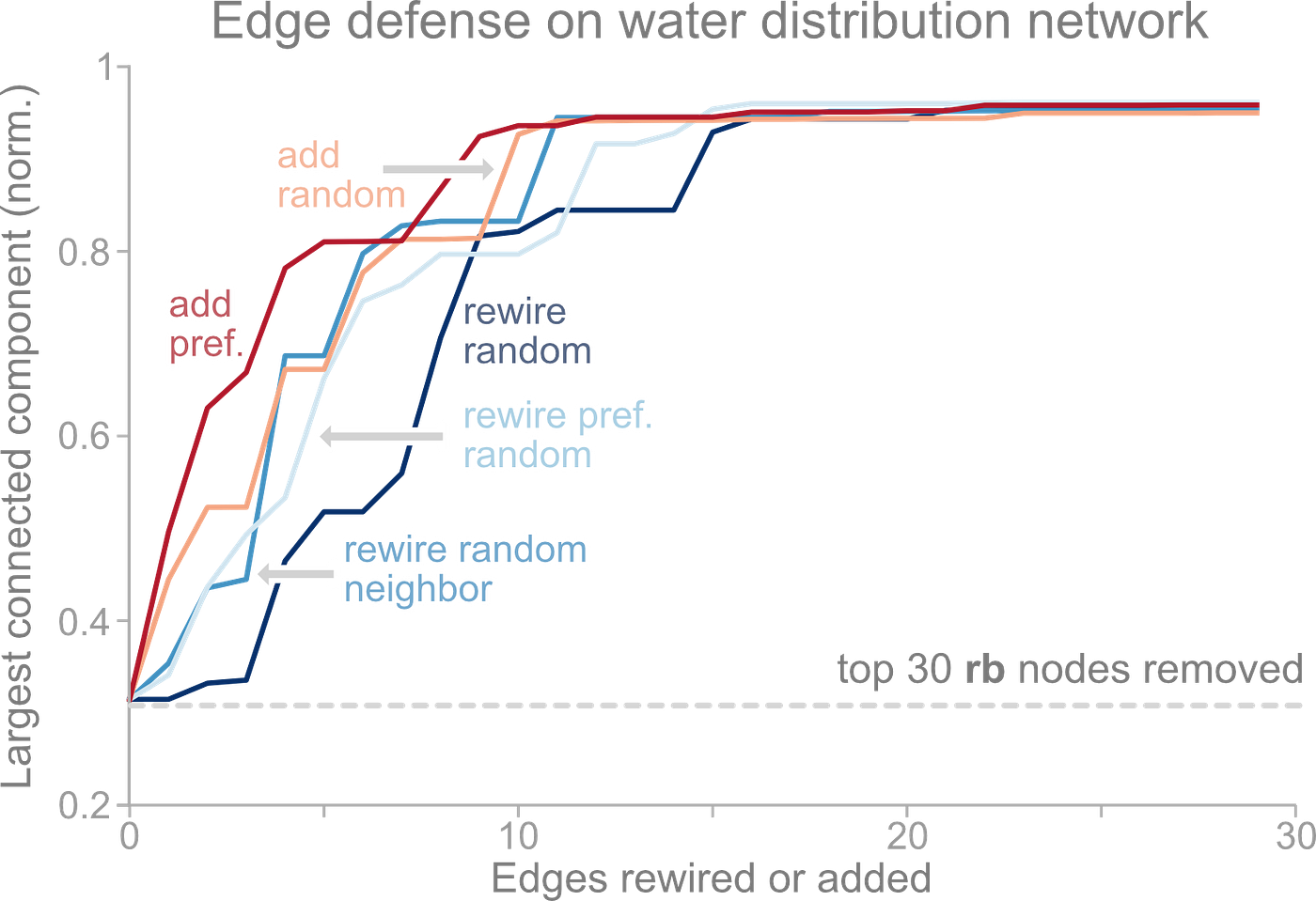Figure 2. Comparing ability of 5 edge defenses to improve KY-2 network robustness after 30 of the most “central” nodes are attacked. Edge addition performs the best, and random edge rewiring performs the worst.

# Network Simulation Tools

TIGER contains 4 broad and important types of robustness simulation tools — (1) dissemination of network entities, (2) cascading failures (3) network attacks, and (4) network defense.

For example, consider Figure 3 which shows an example of a power grid network that is susceptible to both natural failures and targeted attacks. A natural failure occurs when a single power substation fails due to erosion of parts or natural disasters. However, when one substation fails, additional load is routed to alternative substations, potentially causing a series of cascading failures. However, not all failures originate from natural causes, some come from targeted attacks, such as enemy states hacking into the grid to sabotage key equipment to maximally damage the operations of the electrical grid.